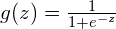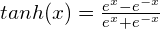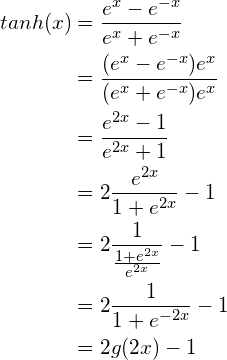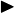# Sigmoid, tanh activations and their loss of popularity

The sigmoid and tanh activation functions were very frequently used for artificial neural networks (ANN) in the past, but they have been losing popularity recently, in the era of Deep Learning. In this blog post, we explore the reasons for this phenomenon.

Definitions

Sigmoid function

Although the original definition of Sigmoid function refers to any functions that have an S-shape in their graphs, a common consensus assumes the formula of Sigmoid function to be equal to the Logistic function, that is:which results in the following graph:

We will stick with this general agreement from now on.

Tanh function

The tanh function is defined as:and graphed as:

In fact, Tanh is just a rescaled and shifted version of the Sigmoid function. We can relate the Tanh function to Sigmoid as below:On a side note, the activation functions that are finite at both ends of their outputs (like Sigmoid and Tanh) are called saturated activation functions (or saturated nonlinearities). The other functions, those who are infinite on at least one of their ends, are non-saturated activation function (or non-saturated nonlinearities).

Their drawbacks

Despise being favored in the past, these 2 activation functions are less used today, especially when it comes to training deep neural networks with a large number of layers.The biggest problem is called Vanishing Gradient. That is, for a deep network with many layers, when we update the weights using backpropagation, the gradients transferred back to the earlier layers get contracted exponentially. At some points, the updating gradients almost vanish (become so close to 0), making the network unable to learn any more.

For example, the derivative of the Sigmoid function, which is:

g'(z) = g(z)(1 – g(z))

(detailed transformation here)

takes the maximum value of 0.25 (when g(z) = 0.5). Imagine you have a 10-layer network, using chain-rule, you would need to multiply at least 10 of those small values to get the gradient for updating the first layer. The final values will likely be squeezed to be tiny, if not vanished.

In fact, you can think of scaling the Sigmoid and Tanh so that their derivatives can take on larger values to overcome this trouble, but then, you jump into a dilemma with the exact opposite issue, called Exploding Gradient – the case when gradients escalate exponentially when being transferred to earlier layers.

From another point of view, most saturated nonlinearities (like Sigmoid and Tanh), even with scaling, are prone to Vanishing Gradient problem, because their gradients at the 2 ends are approximately zero.

The consequence, in this case, is a mix of vanished gradients and exploded gradients, due to the complex multiplication over many layers.The second problem that applies to the Sigmoid activation (but not the Tanh) is being non-zero centered.

Outputs from Sigmoid function are limited in the range (0, 1), meaning they all are always positive. Operating backpropagation, when the gradients flow back to update weights, at a point, if the gradient is positive then all the weights are reduced by some amount, and on the other hand, if the gradient is negative then all the weighted are added by some amount. This uniformity, which is caused by the fact that all weights have the same sign, diminishes the variability and dynamicity of the weights, making the networks learn slower, more zig-zag, and potentially have a lower chance to converge to better states.

The Tanh function, by outputting values in the range (-1, 1), does not suffer this problem, which makes it more frequently used than the Sigmoid in practice.

Are Sigmoid and Tanh coming back?

With the help of normalization techniques, the severity of Vanishing Gradient is somehow lessened.

In their famous paper that introduced Batch Normalization, the authors compare the performance of deep networks with Sigmoid nonlinearity versus ReLU, the standard activation function to date, on the large image dataset LSVRC 2012. It turns out, the accuracy for Sigmoid is just slightly lower than ReLU’s (69.8% vs 73.0%). They also stated that without Batch Normalization, the accuracy of that network (the Inception network) with Sigmoid activation had never exceeded 1/1000.

However, it is too soon to say that Sigmoid and Tanh are coming back, as after 4 years from their return on BatchNorm paper, the ReLU, together with its variations, are still dominating the stage. Sigmoid and Tanh, up to now, rarely, if they really do, appear in state-of-the-art researches.

References: